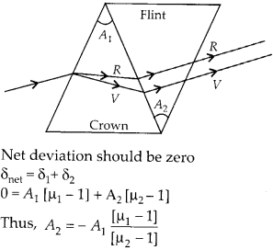# Chapter 9 – Ray Optics and Optical Instruments Questions and Answers: NCERT Solutions for Class 12 Physics

Class 12 Physics NCERT book solutions for Chapter 9 - Ray Optics and Optical Instruments Questions and Answers.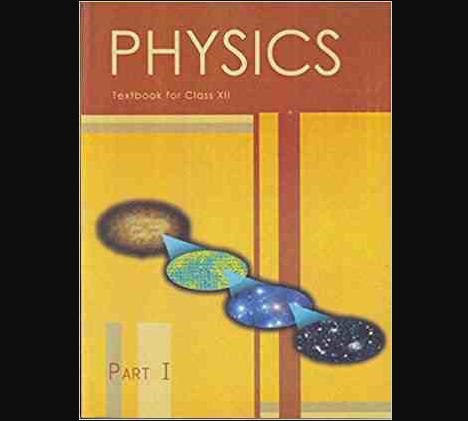## Question 2. A 4.5 cm needle is placed 12 cm away from a convex mirror of focal length 15 cm. Give the location of the image and the magnification. Describe what happens as the needle is moved farther from the mirror.

### Solution: A convex mirror always form virtual image, which is erect and small in size of an object kept in front of it. Focal length of convex mirror ƒ = + 15 cm Object distance u = – 12 cm Using mirror formula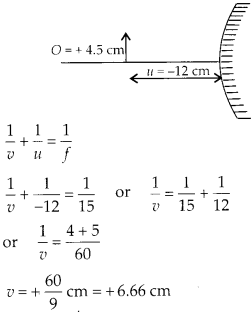##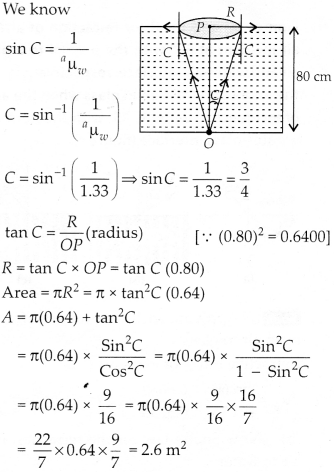Question 6. A prism is made of glass of unknown refractive index. A parallel beam of light is incident on a face of the prism. The angle of minimum deviation is measured to be 40°. What is the refractive index of the material of the prism? The refracting angle of the prism is 60°. If the prism is placed in water (refractive index 1.33), predict the new angle of minimum deviation of a parallel beam of light.

### Solution: When the light beam is incident from air on to the glass prism, the angle of minimum deviation is 40°. Refractive index of glass w.r.t. Air.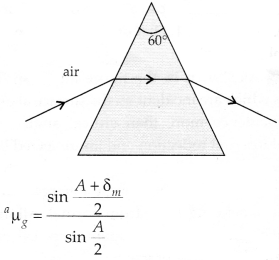## Question 9. An object of size 3.0 cm is placed 14 cm in front of a concave lens of focal length 21 cm. Describe the image produced by the lens. What happens if the object is moved further away from the lens?

### Solution: Object of size 3 cm is placed 14 cm in front of concave lens.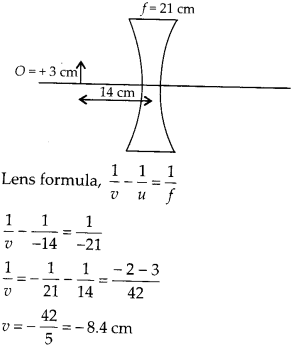## Solution: (a) We want the final image at least distance of distinct vision. Let the object in front of objective is at distance υ0.

###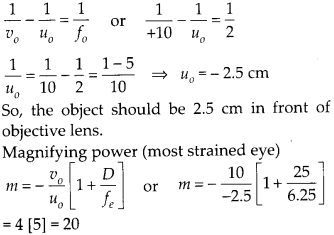(b) We want the final image at infinity. Let us again assume the object in front of objective at distance υ0.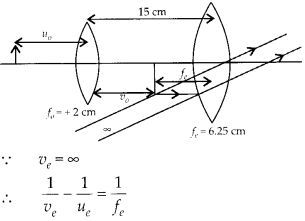##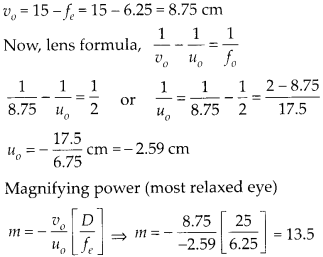Question 12. A person with a normal near point (25 cm) using a compound microscope with objective of focal length 8.0 mm and an eyepiece of focal length 2.5 cm can bring an object placed at 9.0 mm from the objective in sharp focus. What is the separation between the two lenses? Calculate the magnifying power of the microscope.

### Solution: The image is formed at least distance of distinct vision for sharp focus. The separation between two lenses will be υ0 + |υe|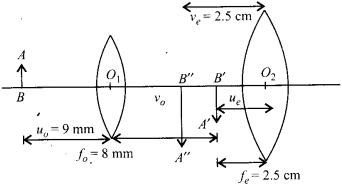##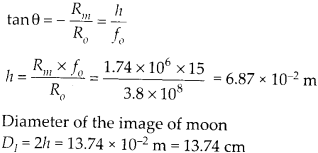Question 15. Use the mirror equation to deduce that: (a) An object placed between f and 2f of a concave mirror produces a real image beyond 2 f. (b) A convex mirror always produces a virtual image independent of the location of the object. (c) The virtual image produced by a convex mirror is always diminished in size and is located Between the focus and the pole. (d) An object placed between the pole and focus of a concave mirror produces a virtual and enlarged image.

###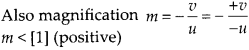So, the image is virtual and diminished. (d) In concave mirror, ƒ < 0 for object placed between focus and pole of concave mirror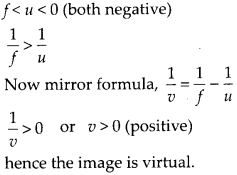##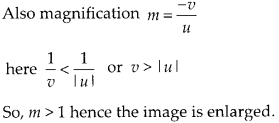Question 16. A small pin fixed on a table top is viewed from above from a distance of 50 cm. By what distance would the pin appear to be raised if it is viewed from the same point through a 15 cm thick glass slab held parallel to the table? Refractive index of glass = 1.5. Does the answer depend on the location of the slab?

### Solution: The shift in the image by the thick glass slab can be calculated. Here, shift only depend upon thickness of glass slab and refractive index of glass. Shift = Real thickness – Apparent of thickness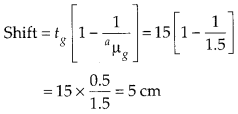## Question 17. (a) Figure shows a cross-section of’light pipe’ made of a glass fiber of refractive index 1.68. The outer covering of the pipe is made of a material of refractive index 1.44. What is the range of the angles of the incident rays with the axis of the pipe for which total reflections inside the pipe take place, as shown in the figure. (b) What is the answer if there is no outer covering of the pipe?

###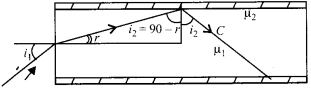(a) Let us first derive the condition for total internal reflection. Critical angle for the interface of medium 1 and medium 2.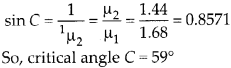### Condition for total internal reflection from core to cladding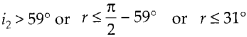###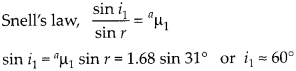Thus all incident rays which makes angle of incidence between 0° and 60° will suffer total internal reflection in the optical fiber. (b) When there is no outer covering critical angle from core to surface.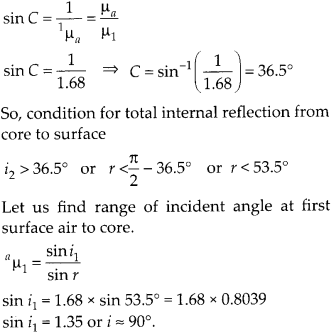## Question 18. Answer the following questions: (a) You have learnt that plane and convex mirrors produce virtual images of objects. Can they produce real images under some circumstances? Explain. (b) A virtual image, we always say, cannot be caught on a screen. Yet when we’see’a virtual image, we are obviously bringing it on to the ‘screen’ (i.e., the retina) of our eye. Is there a contradiction? (c) A diver under water, looks obliquely at a fisherman standing on the bank of a lake. (d) Does the apparent depth of a tank of water change if viewed obliquely? if so, does the apparent depth increase of decrease? (e) The refractive index of diamond is much greater than that of ordinary glass. is this fact of some use to a diamond cutter?

###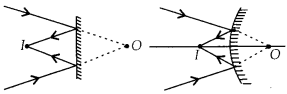(b) Here, the retina is working as a screen, where the rays are converging, but this screen is not at the position of formed virtual image, in fact the reflected divergent rays are converged by the eye lens at retina. Thus, there is no contradiction.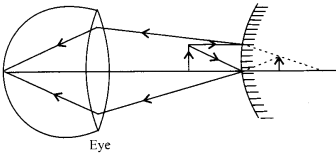## Question 20. A screen is placed 90 cm from an object. The image of the object on the screen is formed by a convex lens at two different locations separated by 20 cm. Determine the focal length of the lens.

### Solution: The image of the object can be located on the screen for two positions of convex lens such that u and v are exchanged. The separation between two positions of the lens is x = 20 cm. It can be observed from figure.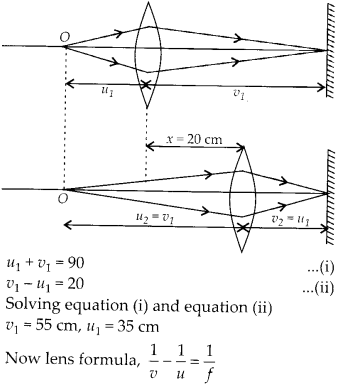##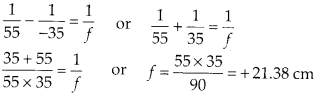Question 21. (a) Determine the ‘effective focal length of the combination of two lenses in question 10, if they are placed 8.0 cm apart with their principal axes coincident. Does the answer depend on which side of the combination a beam of parallel light is incident? Is the notion of effective focal length of this system useful at all? (b) An object 1.5 cm in size is placed on the side of the convex lens in the above arrangement. The distance between the object and the convex lens is 40 cm. Determine the magnification produced by the two-lens system, and the size of the image.

### Solution: (a) (i) Let a parallel beam of light incident first on convex lens, refraction at convex lens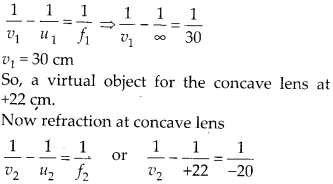###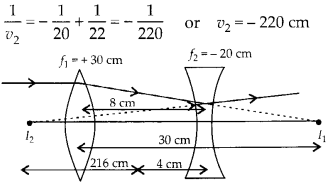The parallel beam of light appears to diverge from a point 216 cm from the center of the two lens system. (ii) Now let a parallel beam of light incident first on concave lens.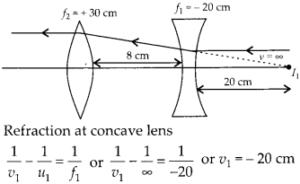###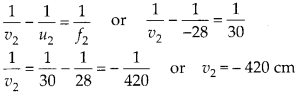Thus the parallel incident beam appears to diverge from a point 420 -4 = 416 cm on the left of the center of the two lens system. Hence the answer depend upon which side of the lens system the parallel beam is made incident. Therefore the effective focal length is different in two situations. (b) Now an object of 1.5 cm size is kept 40 cm in front of convex lens in the same system of lenses.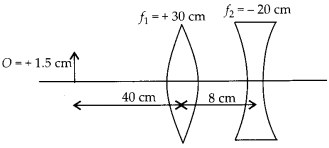##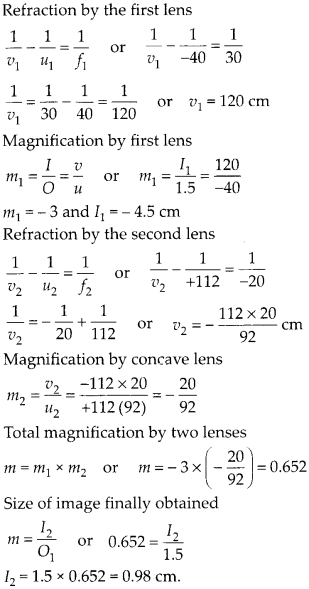Question 22. At what angle should a ray of light be incident on the face of a prism of refracting angle 60° so that it just suffers total internal reflection at the other face? The refractive index of the material of the prism is 1.524.

### Solution: The beam should be incident at critical angle or more than critical angle, for total internal reflection at second surface of the prism.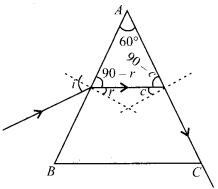##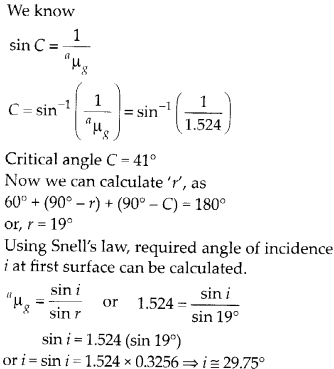Question 23. You are given prisms made of crown glass and flint glass with a wide variety of angles. Suggest a combination of prisms which will (a) deviate a pencil of white light without much dispersion. (b) disperse (and displace) a pencil of white light without much deviation.

### Solution: By using two identical shape prism of crown glass and flint glass kept with their base on opposite sides, we can observe deviation without dispersion or dispersion without deviation. (a) Deviation without dispersion A white beam incident on crown glass will suffer deviation 6, and angular dispersion Δθ, both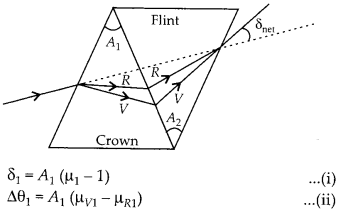### Now the light beam again suffer deviation and dispersion by flint glass prism.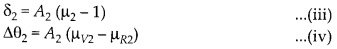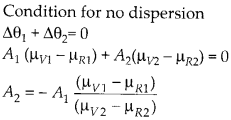### Negative sign shows that two prisms must be placed with their base on opposite sides. Net deviation in this condition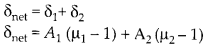### (b) Dispersion without deviation